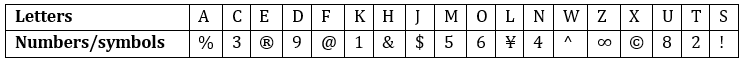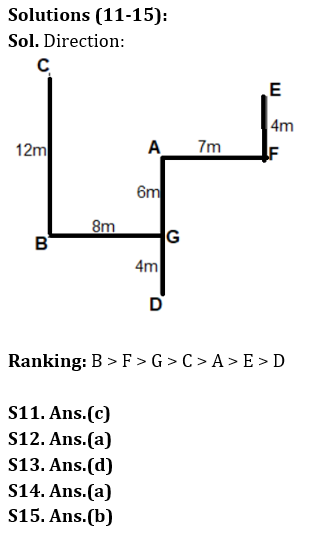Latest Banking jobs   »

# Reasoning Ability Quiz For LIC AAO Mains 2023-16th March

Directions (1-5): In each of the questions given below, a group of letters is given followed by some combinations of number/symbol. You have to find out which of given combination correctly represents the group of letters based on the numbers/symbols codes and the conditions given below. If none of the given combinations represents the group of letters correctly, give (e) i.e. ‘None of these’ as the answer.Condition for coding the group letters:
(i) If the first letter is vowel and last letter is consonant, the codes for the first and the last letter are to be interchanged.
(ii) If the first as well as the last letter is vowel, then both are to be coded by the code for the last letter.
(iii) If the first as well as the last letter is consonant, then both are to be coded by the code for the first letter.
(iv)If the first letter is consonant and last letter is vowel, the codes for the first and the last letter are to be coded as β.

Q1. Find the code for ‘EUWTXO’.
(e) None of these

Q2. Find the code for ‘TOKEHN’.
(a) 261®&2
(b) 216®&2
(c) 261&®2
(d) 26&1®2
(e) None of these

Q3. Find the code for ‘JWXTSA’.
(e) None of these

Q4. Find the code for ‘UMFAWD’.
(a) 95@^%8
(b) 9@%5^8
(c) 95@%^8
(d) 85@%^9
(e) None of these

Q5. Find the code for ‘LEUAOT’.
(a) ¥®8%62
(b) ¥®8%6¥
(c) ¥8®%62
(d) ¥®86%¥
(e) None of these

Directions (6-10): When a number arrangement machine is given an input line of numbers, it arranges them following a particular rule. The following is an illustration of input and rearrangement.

Input: 64533 75894 35467 85434 61346
Step I: 54633 85794 45367 45834 31646
Step II: 85794 45367 45834 54633 31646
Step III: 32579 28536 16583 15463 18164
Step IV: 1079 1536 1283 1063 1064
Step V: 1063 1064 1079 1283 1536
Step VI: 6310 6410 9710 8321 6531

And step VI is the last step of the rearrangement. As per the rules followed in the above step, find out the appropriate steps for the given input.

Input: 23954 77874 15976 89336 75567

Q6. Which of the following is Step III of the given input after the rearrangement?
(a) 32777 35576 18983 54517 36325
(b) 35576 18983 54517 36325 32777
(c) 32777 18983 35576 54517 36325
(d) 32777 35576 54517 18983 36325
(e) None of these

Q7. What is the sum of the highest and the lowest number in step VI of the given input after the rearrangement?
(a) 14052
(b) 14042
(c) 13131
(d) 12942
(e) None of these

Q8. Which of the following combination of numbers, represents the 3rd number from the left end in step V and 2nd number from the right end in step II, respectively of the given input after the rearrangement?
(a) 1277, 87774
(b) 1076, 55767
(c) 1376, 95176
(d) 1225, 93254
(e) None of these

Q9. What is the difference between highest and lowest number in step V of the given input after the rearrangement?
(a) 652
(b) 658
(c) 731
(d) 655
(e) None of these

Q10. Which number is third from the left end in step II?
(a) 39836
(b) 55767
(c) 95176
(d) 87774
(e) None of these

Directions (11-15): Answer the questions based on the information given below:
Seven players A, B, C, D, E, F and G score different runs in a match. Each of them is standing randomly in the playground.
The only player who scores less than E, is 4m south of G. Player who scores the 3rd lowest run is 7m west of the one who scores more than G and C. One of the players is 8m west of G. A is 10m north of D who scores the lowest runs. F is not the highest scorer. C doesn’t score the 3d highest runs. More than three players score more than the one who is 6m north of G. E is 4m north of F. C is 12m north of the highest scorer who is 10m southwest of A.

Q11. What is the direction of the highest run scorer with respect to F?
(a) North-east
(b) North-west
(c) South-west
(d) South-east
(e) None of the above

Q12. Which among the following is to the north-west of the person who scores third highest runs?
(a) C
(b) E
(c) B
(d) D
(e) None of the above

Q13. What is the shortest distance between B and the player who is to the west of F?
(a) 15m
(b) 13m
(c) 20m
(d) 10m
(e) None of the above

Q14. How many players score more than one who is to the east of B?
(a) Two
(b) Three
(c) One
(d) Four
(e) None of the above

Q15. What is the direction of C with respect to D?
(a) North-east
(b) North-west
(c) South-west
(d) South-east
(e) None of the above

Solutions

Solution (1-5):
S1. Ans. (b)
Sol. Condition II is applied.
S2. Ans. (a)
Sol. Condition III is applied.
S3. Ans. (d)
Sol. Condition IV is applied.
S4. Ans. (c)
Sol. Condition I is applied.
S5. Ans. (b)
Sol. Condition III is applied.

Solution (6-10):
Sol. In Step 1: The first three digits in all the numbers from the left end, are written in reverse order with in the number. No change is made to the remaining digits.
Ex-(64533) will be arranged as (54633).
In Step II: The numbers are arranged in descending order from extreme left end (according to the sum of the digits). (Number with the highest sum of digits will be arranged at extreme left end and the number with the 2nd highest sum of digits will be arranged after the 1st number and so on).
In Step III: The product of 1st and last digit from the left end of the number is written (from the left end) at the starting of each number, followed by the remaining digits in the same sequence to form a five-digit number. Ex- (85794) will be arranged as (32579).
(8 × 4) = 32, and (579) will be written in same sequence, so (32579).
In Step IV: The sum of first three digits from the left end is written at the left end of each number within the number, followed by remaining digits in the same sequence to form a four-digit number. Ex- (32579) will be arranged as (1079).
(3+2+5) = 10, and (79) will be written in same sequence, so (1079).
In Step V: All the numbers are arranged in ascending order from the extreme left end.
In Step VI: All the digits of each number are arranged in descending order within the number.

Input: 23954 77874 15976 89336 75567
Step I: 93254 87774 95176 39836 55767
Step II: 87774 55767 39836 95176 93254
Step III: 32777 35576 18983 54517 36325
Step IV: 1277 1376 1883 1417 1225
Step V: 1225 1277 1376 1417 1883
Step VI: 5221 7721 7631 7411 8831

S6. Ans. (a)
S7. Ans. (a)
S8. Ans. (c)
S9. Ans. (b)
S10. Ans. (a)## FAQs

### When will the LIC AAO Mains exam be held?

LIC AAO Mains exam will be held on 18 March

#### Congratulations!Union Budget 2023-24: Free PDF